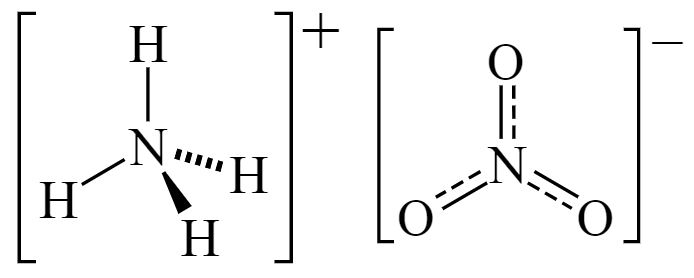# Ammonium Nitrate Formula

Learning about ammonium nitrate formula is crucial as this compound is one of the important inorganic salts in chemistry which also has a lot of applications. Ammonium nitrate is basically a white crystalline powder that is readily soluble in water. It is used in making artificial fertilizers and explosives.

## Ammonium Nitrate Chemical Formula

Ammonium nitrate is a chemical compound made up of two nitrogen atoms, three oxygen atoms and four hydrogen atoms. Therefore the simplified molecular formula of this compound is given as N2H4O3. It also consists of two ions where one is a cation ammonium (NH4+) ion and the other is an anion which is nitrate (NO3) ion. These ions are held together by an ionic bond. It has the chemical formula NH4NO3.

 Formula NH4NO3 Molar Mass 80.043 g/mol Density 1.72 g/cm3 Melting Point 169.6 °C Boiling Point 210 °C

### Ammonium Nitrate Structural Formula

The ammonium nitrate structural formula is represented as;Stay connected to BYJU’S to access all the different formulas of important chemical compounds.